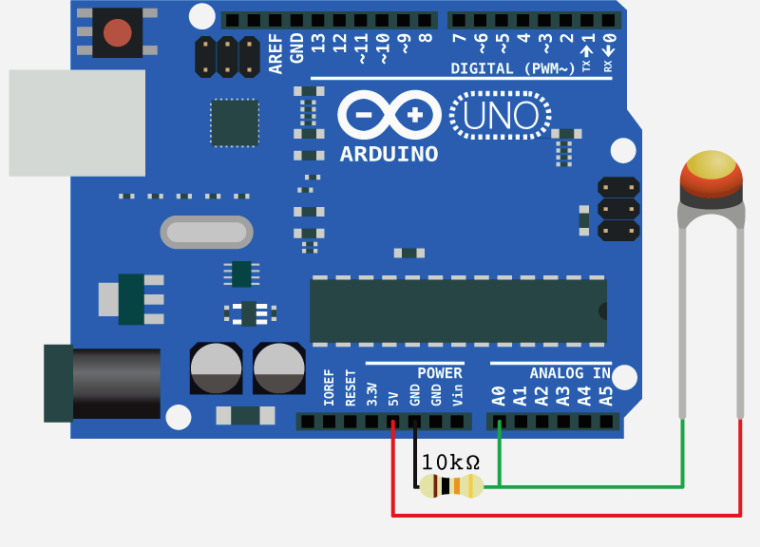# NTC temperature sensor

• @Shaun said in NTC temperature sensor:

apin=adc.channel(attn=3, pin='P16') # should work for you

Great, this changes the bevahiour and looks much better now. Where do I find documentation about this? The official documentation about ADC doesn't talk f.e. about the `attn` parameter.

• @tobru The problem is that the ADC is saturating.
Try change the ADC input attenuation, which will increase the ADC full scale conversion voltage.
attn = (0 = 0db : 1.1V max), (1 = 2.5db : 1.4V max), (2 = 6db : 1.9V max), (3 = 11db : 3.2V max)
apin=adc.channel(attn=3, pin='P16') # should work for you

Edit:
Be warned that the ESP32 ADC is nonlinear and rather noisy.
To reduce the jitter in readings, I take 5 readings at 100us cadence, discard the highest and lowest and average the remaining 3.

The non linearity has to be dealt with using a lookup table or conversion function for now.

• "The 10k resitor" should be the same value as your termistor resistance (it can be different than 10k, I used the one with 50k resistance). This schematic worked for me with esp8266 (on LUA), so I don't think it have a problem with 3.3V

• Ok, looks like the 10K resistor doesn't fit here with 3V3. Nevertheless I'm a bit lost and not sure how to chose the correct resistor and calculate the temperature from the measured value.

• Thanks a lot for the explanation. But I don't get it yet =(

I connected 3V3 to the thermistor on one end, G3 (P16) and the 10k resistor to the other end of the termistor. GND is connected to the 10k resistor (other end).

``````                      10K Thermistor

+-----------+          +-------+
|           |          |       |
|       3V3 +----------+       +----+
|           |          +-------+    |
|           |                       |
|       G3  +-----------------------+
|           |                       |
|           |          +-------+    |
|           |          |       |    |
|       GND +----------+       +----+
+-----------+          +-------+

10K Resistor
``````

All I get is the value 4095 when I read it with the following code:

``````import machine
import time

adc = machine.ADC()
apin = adc.channel(pin='P16')

for i in range(10):
val = apin()
print(val)
time.sleep(1)
``````

What did I understand wrong?

• @tobru said in NTC temperature sensor:

10K NTC

this is simple analog sensor - thermistor
connect it to any analog pin - here you have sample for arduino
but sample is valid for lopy - only difference is that you connect it to 3V3 pin not to 5V pin because pycom board are 3V3 compatibile and not tollerant to 5V

![alt text](image url)

here you have pinout of lopy
https://www.pycom.io/wp-content/uploads/2016/11/lopy_pinout.pdf

and here you have how to program it
https://docs.pycom.io/pycom_esp32/pycom_esp32/tutorial/includes/adc.html

here you have sample scaling table